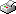Print This Page Boris Lorenc -- licentiate thesis -- Double Samples for Web Surveys and Similar Situations Summary Inference about the general population drawn using unadjusted web survey data is biased if there is a dependence between the study variable and the selection mechanism providing participation in the survey. This work presents two adjustment techniques that may reduce the bias, both based on the double samples procedure, and also studies the effectiveness of the techniques under varying circumstances. With double samples is meant a procedure whereby two samples are taken from the same population, one a random sample on which only auxiliary variables are measured, and another a sample with unknown inclusion probabilities possibly from a subset of the population but on which a more extensive measurement of the study variables is done. One of the techniques, the propensity score weighting, was introduced by George Terhanian (also the originator the double samples procedure), who reported its successful application in the 2000 elections in the USA. Nevertheless, a formal treatment of the technique was never presented, why the first of the studies, "Effectiveness of weighting by stratification on the propensity score using double samples", gives an analytic justification of the technique and illustrates it using a simple trivariate model. While expression for variance of the propensity score adjusted estimator has not been worked out, the variance estimate suggested in the literature is conditional on the model through which the propensity scores are estimated, and was suspected to be underestimating the true variance. Further, while it was known from the analytic demonstration that the adjusted estimator contains a residual bias, it was not known what the bias reducing performance of the estimator would be under varying circumstances regarding for instance correlations of relevant variables and violations of the assumptions on which the technique is laid. To do this, a simulation study was performed, the results of which are reported in "Propensity score weighting with double samples: a simulation study". The second adjustment technique is multiple imputation. It treats the values of the study variables in the random sample---not measured in this sample by design---as missing data, and imputes them using the multiple imputation technique due to Rubin. Amongst the advantages of the technique are the readily available expressions for variance of the point estimator as well as existing software for performing the imputations. In order to study the performance of this technique under the same circumstances as for the propensity score weighting, another simulation study was performed, on which it is reported in "Multiple imputation with double samples: a simulation study".